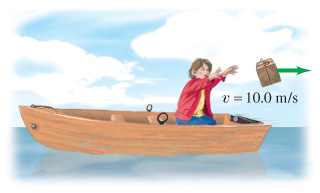Problem: A child in a boat throws a 5.05-kg package out horizontally with a speed of 10.0 m/s. Calculate the velocity of the boat immediately after, assuming it was initially at rest. The mass of the child is 26.0 kg and that of the boat is 45.0 kg. (Take the packages direction of motion as positive.)

FREE Expert Solution
96% (240 ratings)
Problem Details

A child in a boat throws a 5.05-kg package out horizontally with a speed of 10.0 m/s. Calculate the velocity of the boat immediately after, assuming it was initially at rest. The mass of the child is 26.0 kg and that of the boat is 45.0 kg. (Take the packages direction of motion as positive.)